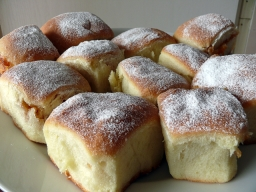# Quarter 26363

There were buns in the basket on the table. Josef ate half of the half, Eva ate the rest, Katka ate two buns, and Dunčo ate a quarter.
How many buns were on the table?

x =  16

### Step-by-step explanation:

x/2+(x-x/2)/4+2+x/4=x

0.5x = 8

x = 8/0.5 = 16

x = 16

Our simple equation calculator calculates it.Did you find an error or inaccuracy? Feel free to write us. Thank you!

Tips for related online calculators
Need help calculating sum, simplifying, or multiplying fractions? Try our fraction calculator.
Do you have a linear equation or system of equations and looking for its solution? Or do you have a quadratic equation?